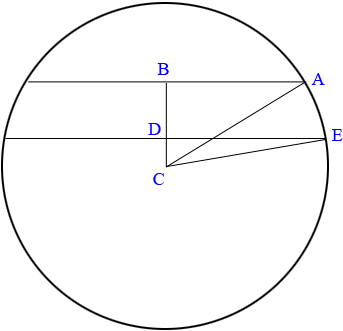SEARCH HOMEMath Central Quandaries & QueriesQuestion from Don: two parallel chords of a circle AB and CD on the same side from the center measure 6cm and 11 cm respectively. The distance between the 2 chords is 3 cm. Find the radiusHi Don,

Here is my diagram but I changed the labeling.C is the centre of the circle. Triangles ABC and EDC are both right triangles. Suppose the distance from C to D is $x$ and the radius of the circle is $r.$ You know the lengths of AB, ED and BD.

Use Pythagoras' theorem and the triangle EDC to find an expression for $r^2$. Use Pythagoras' theorem and the triangle ABD to find another expression for $r^2$. Set theses expression equal to each other and solve for $x.$

PennyMath Central is supported by the University of Regina and The Pacific Institute for the Mathematical Sciences.